Bala Venkateshbalavenkatesh.s

11min

# How Does the Machine Read Images and Use them in Computer Vision?

Before we look deeply into how machines read images and use them in computer vision, it’s important to understand how we can read and store images in machines. This is especially key if we are working on computer vision applications.

We are also going to study how we can read images in machines using Python. Python provides a lot of flexibility to use functions like cropping, resizing and blurring using OpenCV or Pillow library, which is built by Python.

### Introduction

As an example let’s look at the image below.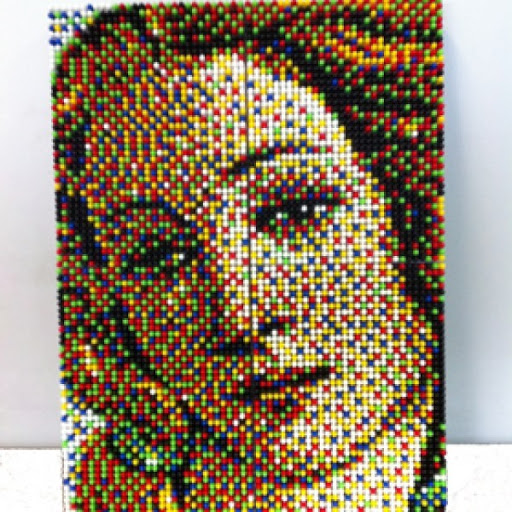We have an image of a girl. Look closely and you will notice that it is made up of small square boxes. These are called pixels. There are some limitations, as we see the images in their visual form, we as humans can easily differentiate the edges and colors. Unlike us, machines can’t easily identify those. Instead, machines store images in the form of numbers.

Lets see the image below to understand this concept more clearly: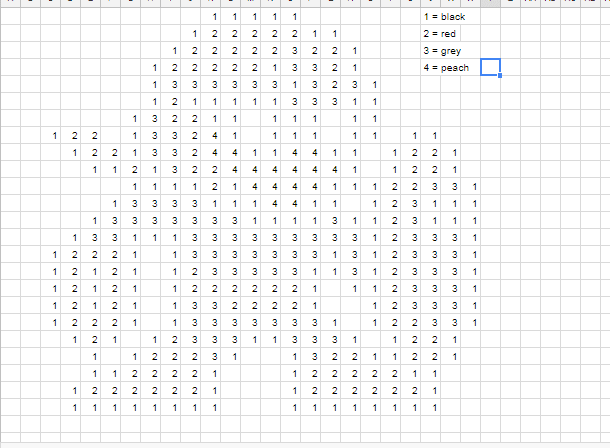The above shows how the machine stores images with particular codes. The machine stores them numerically as “1” which indicates RED, “2” which indicates GREEN, and “3” which indicates BLUE. Similarly, colored images are stored in 3D matrices and grayscale images are stored in 1D matrices.

How to change colored images to grayscale images using Python code:

Example code:

``````1
2
3
4
5
6
7
8
``````from PIL
import Image, ImageOps

im1 = Image.open(r "balavenkatesh.JPG")

im2 = ImageOps.grayscale(im1)

im2.show()
``````

A colored image is composed of multiple colors and all colors can be generated from three (red, green and blue) colors.

The color range between 0-255 represents the intensity of the color for that pixel.That concept is illustrated here: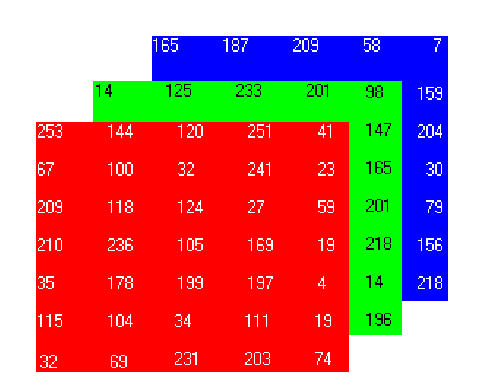We have a colored image and we have three matrices for the red, green, and blue (RGB) channels.

This is not an original pixel value for the given image because the original matrix would be very large and take a lot of memory to store in the computer.

Also, it would be a little difficult to visualize the matrix form. There are various formats in which the image can be stored. RGB is the popular one, so I have described it here. If you want to learn about the other image formats check them out here.

### Gray Pixel Values as Features

Consider the same example for our image below (‘8’). The dimension of the image is 28 x 28.

The number of features will be the same as the number of pixels. Hence, that number will be 784.

Now here’s another curious question – how do we arrange these 784 pixels as features? Well, we can simply append every pixel value one after the other to generate a feature vector.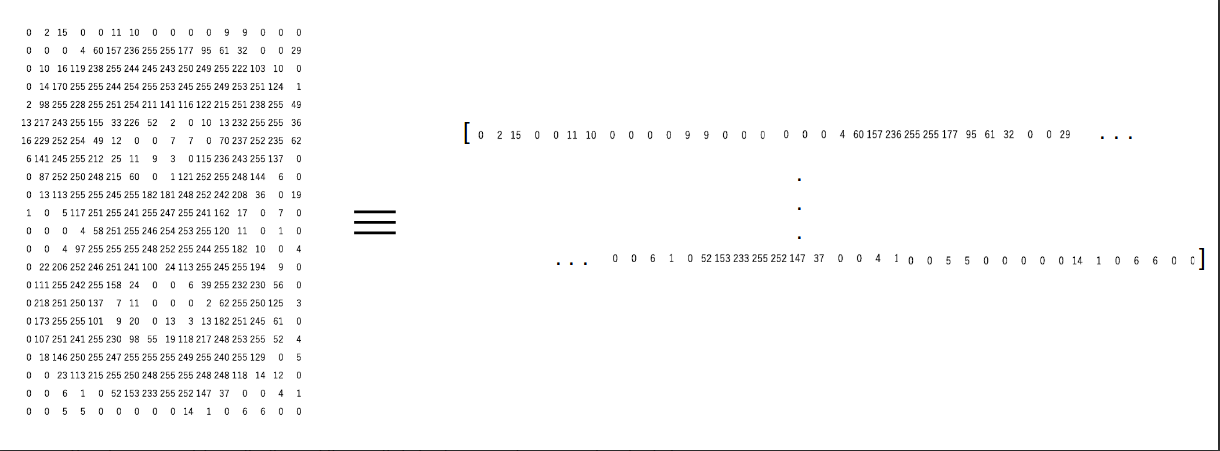Example code:

``````1
2
3
4
5
6
7
8
9
10
11
12
13
14
``````image = imread('topoder.jpeg', as_gray = True)

image.shape, imshow(image)

The image shape here is 650 x 450. Hence, the number of features should be 297, 000. We can generate this using the reshape
function from NumPy where we specify the dimension of the image:

features = np.reshape(image, (660 * 450))

print(features.shape, features)

(297000, )

array([0.96470588, 0.96470588, 0.96470588, ..., 0.96862745, 0.96470588, 0.96470588])
``````

### How to Apply Mean Pixel Value of a Channel in an Image

Mean pixel value converts a 3D matrix to a 1D matrix. Instead of getting all pixel values from three channels (RGB) separately, we have to generate a new matrix which has the mean value of pixels from all three channels.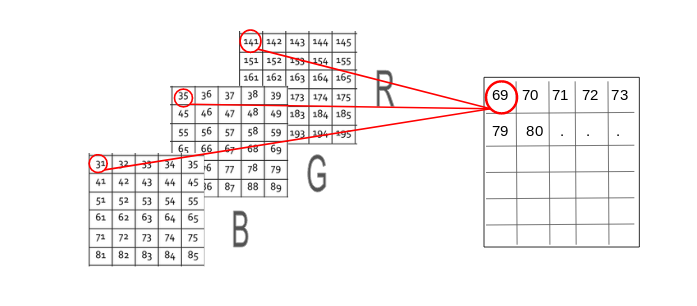We will use Python code to get mean average values.

``````1
2
3
``````for i in range(0, image.shape):
for j in range(0, image.shape):
feature_matrix[i][j] = ((int(image[i, j, 0]) + int(image[i, j, 1]) + int(image[i, j, 2])) / 3)
``````

We have a 3D matrix of dimension (360 x 250 x 3) where 360 is the height, 250 is the width and 3 is the number of channels.

As you can see in the above image, the new matrix will have the same height and width but  one channel only. Now we can get all mean values from the three matrices and create new matrices.

``````1
2
``````features = np.reshape(feature_matrix, (360 * 250))
features.shape(90000, )
``````

### Extracting Edge Features

Consider that we are given the below image and we need to identify the objects in it:You must have recognized the object as the car. What are the features that you considered while differentiating this image? The shape could be one important factor, followed by color, or size. What if the machine could also identify the shape as we do?

A similar idea is to extract edges as features and use that as the input for the model. I want you to think about this for a moment – how can we identify edges in an image? An edge is basically where there is a sharp change in color. Look at the below image: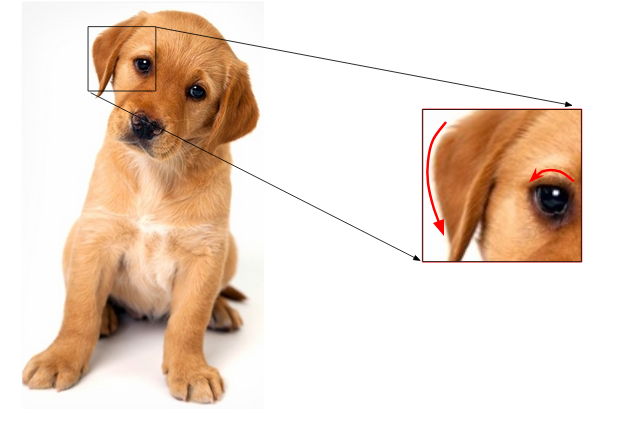I have highlighted two edges here. We could identify the edge because there was a change in color from white to brown (in the right image) and brown to black (in the left). And as we know, an image is represented in the form of numbers. So, we will look for pixels around which there is a drastic change in the pixel values.

Let’s say we have the following matrix for the image: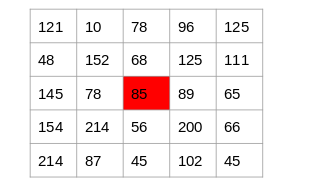To identify if a pixel is an edge or not, we will simply subtract the values on either side of the pixel. For this example, we have the highlighted value of 85. We will find the difference between the values 89 and 78. Since this difference is not very large, we can say that there is no edge around this pixel.

Now consider the pixel 125 highlighted in the below image: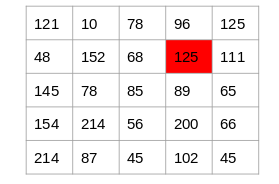Since the difference between the values on either side of this pixel is large, we can conclude that there is a significant transition at this pixel and hence it is an edge.

### Conclusion

I believe this article gives some fundamentals about storing images in computers. I would like to also add that every Computer Vision Engineer should know this concept.